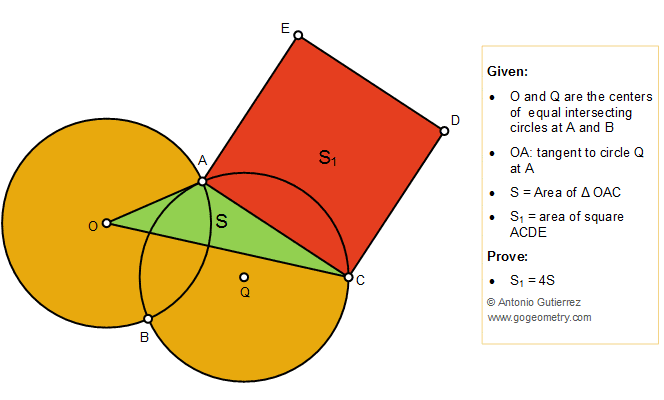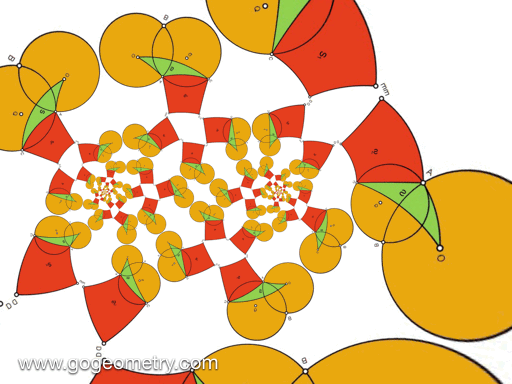# Geometry Problem 1428: Intersecting Circles, Tangent Line, Triangle, Square, Area

As shown in the figure below, O and Q are the centers of equal intersecting circles at A and B so that OA is tangent to circle Q at A. If S is the area of the triangle OAC and S1 is the area of the square ACDE, prove that S1 = 4S### Geometric Art of Problem 1428: Sketching, Typography, iPad Apps### Animation of the Conformal Mapping or Transformation of Problem 1428### Conformal Mapping

A conformal mapping or conformal transformation is a continuous mapping preserving the form of infinitesimal figures. This conformal map produces a realistic view of the original image or map. This the conformal transformation of problem1428.